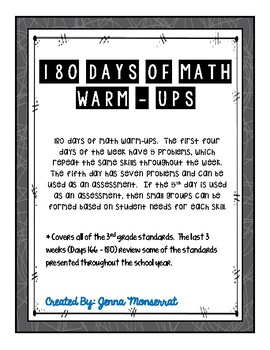# Math Warm-UpsSubject
Resource Type
File Type

PDF

(6 MB)
Standards
• Product Description
• StandardsNEW

A whole year of math warm-ups. Each day has 5 problems, except the 5th day, which has 7. Every day has a subtraction with regrouping problem and a word problem. The other three problems are based on the 3rd grade standards. Great to use for students to independently to get started with their math block. The 5th day can be used as a quick assessment to check for understanding of the standards.

Recognize area as additive. Find areas of rectilinear figures by decomposing them into non-overlapping rectangles and adding the areas of the non-overlapping parts, applying this technique to solve real world problems.
Use tiling to show in a concrete case that the area of a rectangle with whole-number side lengths 𝘢 and 𝘣 + 𝘤 is the sum of 𝘢 × 𝘣 and 𝘢 × 𝘤. Use area models to represent the distributive property in mathematical reasoning.
Partition shapes into parts with equal areas. Express the area of each part as a unit fraction of the whole. For example, partition a shape into 4 parts with equal area, and describe the area of each part as 1/4 of the area of the shape.
Understand that shapes in different categories (e.g., rhombuses, rectangles, and others) may share attributes (e.g., having four sides), and that the shared attributes can define a larger category (e.g., quadrilaterals). Recognize rhombuses, rectangles, and squares as examples of quadrilaterals, and draw examples of quadrilaterals that do not belong to any of these subcategories.
Compare two fractions with the same numerator or the same denominator by reasoning about their size. Recognize that comparisons are valid only when the two fractions refer to the same whole. Record the results of comparisons with the symbols >, =, or <, and justify the conclusions, e.g., by using a visual fraction model.
Total Pages
N/A
N/A
Teaching Duration
N/A
Report this Resource to TpT
Reported resources will be reviewed by our team. Report this resource to let us know if this resource violates TpT’s content guidelines.